Next: Compatible Observables Up: Fundamental Concepts Previous: Expectation Values

# Degeneracy

Suppose that two different (normalized) eigenstates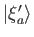and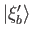of the observable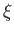correspond to the same eigenvalue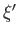. These states are termed degenerate eigenstates. Degenerate eigenstates are necessarily orthogonal to any eigenstates corresponding to different eigenvalues, but, in general, they are not orthogonal to each other (i.e., the proof of orthogonality given in Section 1.8 does not work in this case). This is unfortunate, because much of the previous formalism depends crucially on the mutual orthogonality of the different eigenstates of an observable. Note, however, that any linear combination ofandis also an eigenstate corresponding to the eigenvalue. It follows that we can always construct two mutually orthogonal degenerate (normalized) eigenstates. For instance,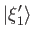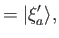(1.64)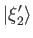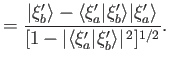(1.65)

This result is easily generalized to the case of more than two degenerate eigenstates. (See Exercises 10.) We conclude that it is always possible to construct a complete set of mutually orthogonal eigenstates for any given observable.Next: Compatible Observables Up: Fundamental Concepts Previous: Expectation Values
Richard Fitzpatrick 2016-01-22